Test: Solid Mechanics- 1

# Test: Solid Mechanics- 1

Test Description

## 10 Questions MCQ Test GATE Civil Engineering (CE) 2023 Mock Test Series | Test: Solid Mechanics- 1

Test: Solid Mechanics- 1 for Civil Engineering (CE) 2023 is part of GATE Civil Engineering (CE) 2023 Mock Test Series preparation. The Test: Solid Mechanics- 1 questions and answers have been prepared according to the Civil Engineering (CE) exam syllabus.The Test: Solid Mechanics- 1 MCQs are made for Civil Engineering (CE) 2023 Exam. Find important definitions, questions, notes, meanings, examples, exercises, MCQs and online tests for Test: Solid Mechanics- 1 below.
Solutions of Test: Solid Mechanics- 1 questions in English are available as part of our GATE Civil Engineering (CE) 2023 Mock Test Series for Civil Engineering (CE) & Test: Solid Mechanics- 1 solutions in Hindi for GATE Civil Engineering (CE) 2023 Mock Test Series course. Download more important topics, notes, lectures and mock test series for Civil Engineering (CE) Exam by signing up for free. Attempt Test: Solid Mechanics- 1 | 10 questions in 30 minutes | Mock test for Civil Engineering (CE) preparation | Free important questions MCQ to study GATE Civil Engineering (CE) 2023 Mock Test Series for Civil Engineering (CE) Exam | Download free PDF with solutions
 1 Crore+ students have signed up on EduRev. Have you?
Test: Solid Mechanics- 1 - Question 1

### In the tension test of a bar the fractured surface shows a truncated cone, the material may be:

Detailed Solution for Test: Solid Mechanics- 1 - Question 1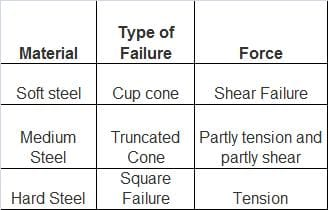Test: Solid Mechanics- 1 - Question 2

### In the engineering stress-strain curve for mild steel, the Ultimate Tensile Strength (UTS) refers to

Detailed Solution for Test: Solid Mechanics- 1 - Question 2

The engineering stress-strain curve for mild steel is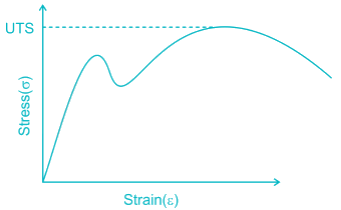Ultimate tensile strength represents the maximum stress that a material can withstand without Fracture.

Test: Solid Mechanics- 1 - Question 3

### _______ is the capacity of material to absorb energy when it is elastically deformed and then upon unloading, to have this energy recovered.

Detailed Solution for Test: Solid Mechanics- 1 - Question 3

Resilience: It is energy absorbed by a member in elastic region. It denotes the capacity of material to absorb energy when it is elastically deformed and then upon unloading, to release this energy.

Toughness: It is energy absorbed by member just before its fracture.

Test: Solid Mechanics- 1 - Question 4

The shear modulus of a material is half of its Young’s modulus. What is the value of its Poisson’s ratio?

Detailed Solution for Test: Solid Mechanics- 1 - Question 4

It is given that the shear modulus is half of Young's modulus and the relationship between shear modulus (G) and poisson's ratio (E) is given as follows: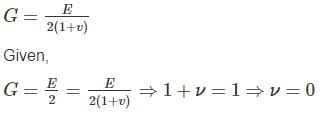Test: Solid Mechanics- 1 - Question 5

A mild steel wire is 10 mm in diameter and 1 m long. If the wire is subjected to an axial tensile load 10 kN, find an extension of the road (Take E = 200 × 10Pa) :

Detailed Solution for Test: Solid Mechanics- 1 - Question 5

The formula for extension is given as follows where, P = load , L = length, A = area and E = 200 x 109 Pa. Substituting values of P,L,A and E.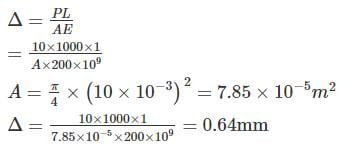Test: Solid Mechanics- 1 - Question 6

The ratio of strengths of solid to hollow shafts, both having outside diameter D and hollow having inside diameter D/2, in torsion, is

Detailed Solution for Test: Solid Mechanics- 1 - Question 6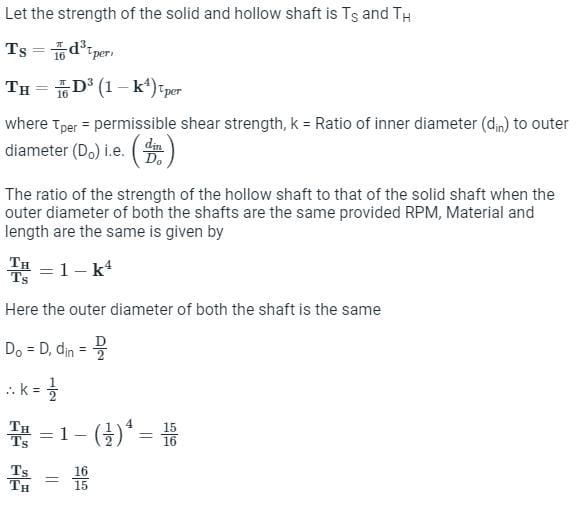Test: Solid Mechanics- 1 - Question 7

A simply supported beam is subjected to a uniformly distributed load. Which one of the following statements is true?

Detailed Solution for Test: Solid Mechanics- 1 - Question 7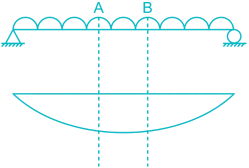For section AB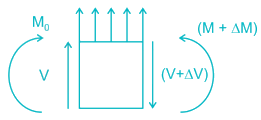For equilibrium, ∑M0 = 0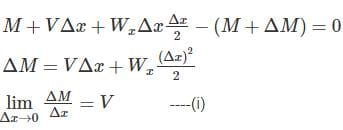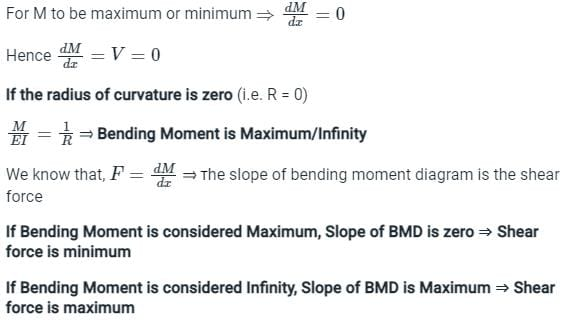Test: Solid Mechanics- 1 - Question 8

A steel plate of side ‘a’ (2d) is enclosed in a closely fitting rigid support as shown. Assume friction between plate and support is zero. Determine the free expansion in x - direction of the plate due to heating by ΔT – (Poison ratio is μ & thermal co - eff. α)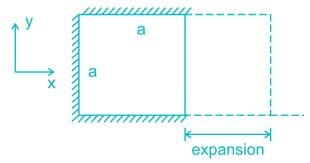Detailed Solution for Test: Solid Mechanics- 1 - Question 8

σx = 0
σy = −αTE
εy = −αT
εx = −με= μαT = δa/a
ϵx = Free expansion due to temperature change + Expansion due to lateral strain

= a α Δ T + μ a α Δ T (due to y direction)

= a α Δ T (1 + μ)

*Answer can only contain numeric values
Test: Solid Mechanics- 1 - Question 9

For a cylindrical bar of 30 mm dia& 900 mm length, during a tension test, it is found that longitudinal strain is 4 times of lateral strain. The bulk modulus for the bar is (X) × 105 N/mm2  (if E = 3 × 105 N/mm2) The value if X is ___________

Detailed Solution for Test: Solid Mechanics- 1 - Question 9

E = 3 × 105 N/mm2 & E = 3k (1 – 2μ).....(1)
From (1) we get K = E/3(1−2μ) [∴μ=0.25 given]
put μ=0.25 in equation 1,we get
k=2*105N/mm2.

Test: Solid Mechanics- 1 - Question 10

A cantilever beam has the cross-section of an isosceles triangle and is loaded as shown in figure. If the moment of inertia of the cross-section Izz = 1/36m4, then the maximum bending stress is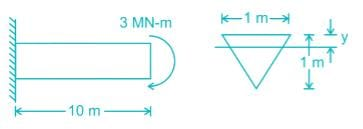Detailed Solution for Test: Solid Mechanics- 1 - Question 10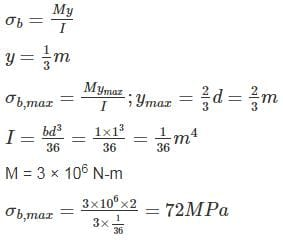## GATE Civil Engineering (CE) 2023 Mock Test Series

26 docs|292 tests
 Use Code STAYHOME200 and get INR 200 additional OFF Use Coupon Code
Information about Test: Solid Mechanics- 1 Page
In this test you can find the Exam questions for Test: Solid Mechanics- 1 solved & explained in the simplest way possible. Besides giving Questions and answers for Test: Solid Mechanics- 1, EduRev gives you an ample number of Online tests for practice

## GATE Civil Engineering (CE) 2023 Mock Test Series

26 docs|292 tests